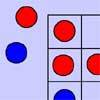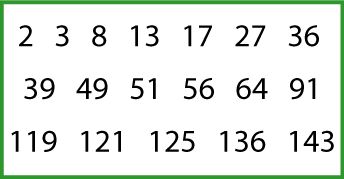#### You may also like### Number Detective

Follow the clues to find the mystery number.### Red Even

You have 4 red and 5 blue counters. How many ways can they be placed on a 3 by 3 grid so that all the rows columns and diagonals have an even number of red counters?### Prime Magic

Place the numbers 1, 2, 3,..., 9 one on each square of a 3 by 3 grid so that all the rows and columns add up to a prime number. How many different solutions can you find?

# Sets of Numbers

## Sets of Numbers

How many different sets of numbers with at least four members can you find in the numbers in this box?For example, one set could be multiples of $4$ {$8, 36 ...$}, another could be odd numbers {$3, 13 ...$}.

### Why do this problem?

This problem gives plenty of opportunity for both discussion and exploring patterns, properties and relationships involving numbers. It also helps learners to make general statements about properties of numbers such as squares, factors, multiples, odd and even, and to identify examples for which a statement is true or false.

### Possible approach

You could also use this interactivity as a starter to this problem where you drag numbers you "like" (i.e. are part of a set) to one side and numbers you "don't like" (i.e. are not in your set) to the other. The children then have to ask questions with yes/no answers to determine the name of your set. You could invite some learners to take the lead on this themselves, with the rest of the group asking questions.

You could then introduce the problem itself and learners could work in pairs from this sheet so that they are able to talk through their ideas with a partner. (The sheet has two copies of the box of numbers on it.) You could also challenge pairs to find two other possible members for their sets from the numbers under $150$.

At the end learners could be asked for the titles of the sets they have found and then others could suggest members for these sets. You could make statements such as "$9$ is a member of this set" and ask learners to identify whether the statement is true or false. When sets of multiples of $2$, $4$ and $8$ have been found and discussed, learners could be asked to explain why no other sets of multiples of even numbers are possible from the numbers in the box.